# High School Math : Function Properties

## Example Questions

### Example Question #1 : Derivatives

The functionis such that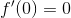When you take the second derivative of the function, you obtain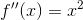What can you conclude about the function at?

The point is an absolute maximum.

The point is a local maximum.

The point is a local minimum.

The point is an absolute minimum.

The point is an inflection point.

The point is an inflection point.

Explanation:

We have a point at which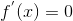. We know from the second derivative test that if the second derivative is negative, the function has a maximum at that point. If the second derivative is positive, the function has a minimum at that point. If the second derivative is zero, the function has an inflection point at that point.

Plug in 0 into the second derivative to obtain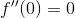So the point is an inflection point.

### Example Question #2 : Derivatives

Consider the function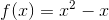Find the maximum of the function on the interval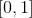.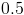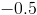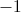Explanation:

Notice that on the interval, the termis always less than or equal to. So the function is largest at the points when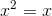. This occurs atand.

Plugging in either 1 or 0 into the original functionyields the correct answer of 0.

### Example Question #1 : Relations And Functions

What is the domain of the function below: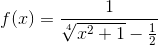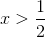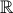Explanation:

The domain is defined as the set of possible values for the x variable. In order to find the impossible values of x, we should:

a) Set the equation under the radical equal to zero and look for probable x values that make the expression inside the radical negative: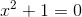There is no real value for x that will fit this equation, because any real value square is a positive number i.e. cannot be a negative number.

b) Set the denominator of the fractional function equal to zero and look for probable x values: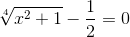Now we can solve the equation for x: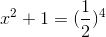There is no real value for x that will fit this equation.

The radical is always positive and denominator is never equal to zero, so the f(x) is defined for all real values of x. That means the set of all real numbers is the domain of the f(x) and the correct answer is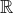.

Alternative solution for the second part of the solution:

After figuring out that the expression under the radical is always positive (part a), we can solve the radical and therefore denominator for the least possible value (minimum value). Setting the x value equal to zero will give the minimum possible value for the denominator.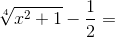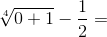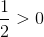That means the denominator will always be a positive value greater than 1/2; thus it cannot be equal to zero by setting any real value for x. Therefore the set of all real numbers is the domain of the f(x).

### Example Question #1 : Pre Calculus

What is the domain of the function below?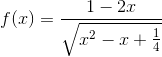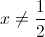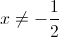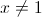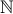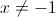Explanation:

The domain is defined as the set of all values of x for which the function is defined i.e. has a real result. The square root of a negative number isn't defined, so we should find the intervals where that occurs: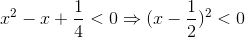The square of any number is positive, so we can't eliminate any x-values yet.

If the denominator is zero, the expression will also be undefined.

Find the x-values which would make the denominator 0: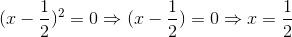Therefore, the domain is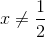.

### Example Question #1 : Relations And Functions

What is the domain of the function below: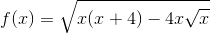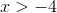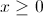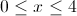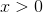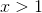Explanation:

The domain is defined as the set of all possible values of the independent variable. First,must be greater than or equal to zero, because if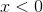, then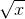will be undefined. In addition, the total expression under the radical, i.e.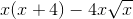must be greater than or equal to zero: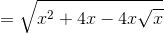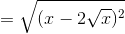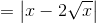That means that the expression under the radical is always positive and thereforeis defined. Hence, the domain of the functionis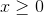.

### Example Question #1 : Function Properties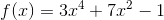This function is:

not symmetric

Explanation:

A function's symmetry is related to its classification as even, odd, or neither.

Even functions obey the following rule: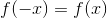Because of this, even functions are symmetric about the y-axis.

Odd functions obey the following rule: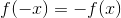Because of this, odd functions are symmetric about the origin.

If a function does not obey either rule, it is neither odd nor even. (A graph that is symmetric about the x-axis is not a function, because it does not pass the vertical line test.)

To test for symmetry, simply substitute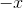into the original equation.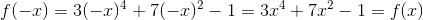Thus, this equation is even and therefore symmetric about the y-axis.

### Example Question #1 : Finding Asymptotes

A function is defined by the following rational equation: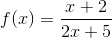What are the horizontal and vertical asymptotes of this function's graph?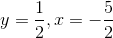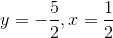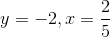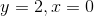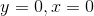Explanation:

To find the horizontal asymptote, compare the degrees of the top and bottom polynomials. In this case, the two degrees are the same (1), which means that the equation of the horizontal asymptote is equal to the ratio of the leading coefficients (top : bottom). Since the numerator's leading coefficient is 1, and the denominator's leading coefficient is 2, the equation of the horizontal asymptote is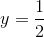.

To find the vertical asymptote, set the denominator equal to zero to find when the entire function is undefined: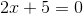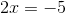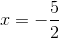### Example Question #2 : Finding Asymptotes

A function is defined by the following rational equation: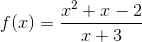What line doesapproach asapproaches infinity?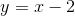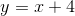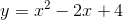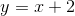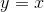Explanation:

This question is asking for the equation's slant asymptote. To find the slant asymptote, divide the numerator by the denominator. Long division gives us the following: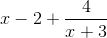However, because we are consideringas it approaches infinity, the effect that the last term has on the overall linear equation quickly becomes negligible (tends to zero). Thus, it can be ignored.

### All High School Math Resources